# Object Orientation

Here we are going to discuss the concept of lagging and leading scheme on DNA System using four-dimensional space (4D). A mathematical extension of the concept of three-dimensional space (3D) originated by 43 out of 89 objects of bilateral 9 sums.

``````---+-----+-----
1 | {1} |{43}
---+-----+-----
2 | 44  |{57}
---+-----+-----
3 | 58  | 59
---+-----+-----
4 | 60  | 104
---+-----+-----
5 | 105 |{115}
---+-----+-----
6 |{116}| 134
---+-----+-----
7 | 135 | 162
---+-----+-----
8 | 163 | 175
---+-----+-----
9 | 176 |{176}
---+-----+-----
``````

The geometry of four-dimensional space is much more complex than that of three-dimensional space, due to the extra degree of freedom. However in our case this 43 objects has excatly a finite fraction of four (4) axis dimensions to MEC30.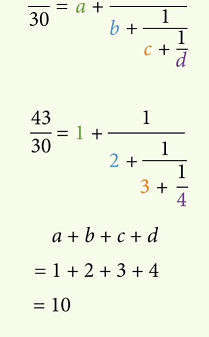(114/2)! = 57! = 1653 » 1653 / 57 = 29

``````--------+
| ⅓
+---   } ⅔
Case A | ⅓
+---------
| ⅓      |
-----------------+  Φ = ⅔
| ⅓      |
+---------
Case B | ⅓
+---   } ⅔
| ⅓
---------
``````

9 + 19 + 29 = 28 + 29 = 57

``````P7:(142857)

#  |  A   |  B   | ∑
------+------+------+-----
{1} |      |      |
------+      |      |
...  |  28  |  29  | 57
------+      |      |
{57} |      |      |
------+------+------+-----
58  |      |      |
------+      |      |
... |  29  |  28  | 57
------+      |      |
114  |      |      |
------+------+------+-----
|  57  |  57  | 114
``````

Comparatively, four-dimensional space has an extra coordinate axis, orthogonal to the other three, which is usually labeled w to describe the two additional cardinal directions of up toward and down from, respectively.

The set of points in Euclidean 4-space having the same distance R from a fixed point P0 forms a hypersurface known as a 3-sphere where R is substituted by function R(t) with t meaning the cosmological age of the universe. Growing or shrinking R with time means expanding or collapsing universe, depending on the mass density inside (Wikipedia).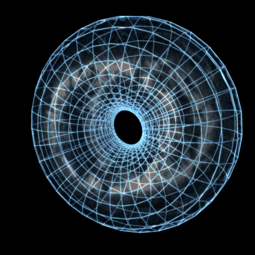By deploying containers on Compute Engine, you can simplify app deployment while controlling four dimensional space. You can configure a virtual machine (VM) instance or an instance template to deploy and launch a Docker container.

## Balanced Prime

The initial objects will be a formation of a double helix driven by vectors 71 and 68 based on the arrangement of prime numbers on a cube of 10x10x10 or 1000 to the Golden Ratio.

π(10x10x10) + 10x10x10/Φ = π(1000) + 1000/Φ = 168 + 618 = (7x71) + (17x17) = 786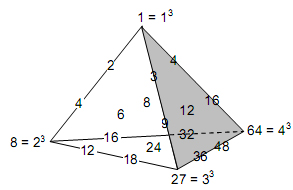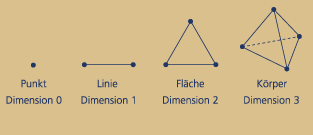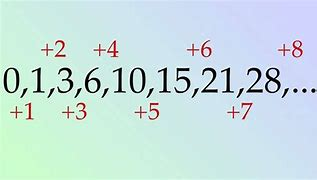1/7 = 0,142857142857142857142857.. infinity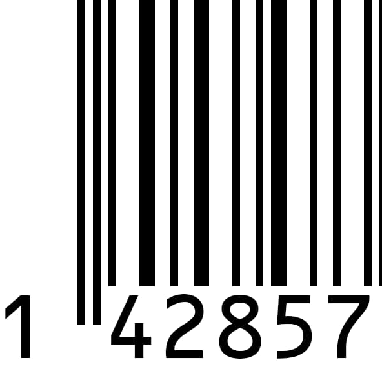By the prime numbers this polarizing between the objects and its tensors is identified by the 157 as the 19+18=37th prime. This 157 is a balanced prime of two (2) primes of 151 + 163 = 314 = 100 x π by which the 100 is standing as the square of 10x10 out of the central objects of ten (10) while the π is one of the constant of Euler's identity.

The number 157 is the 18+19=37th prime number, a balanced prime, because the arithmetic mean of those primes yields 157. The next prime is 163 and the previous prime is 151, with which 157 forms a prime triplet (Wikipedia).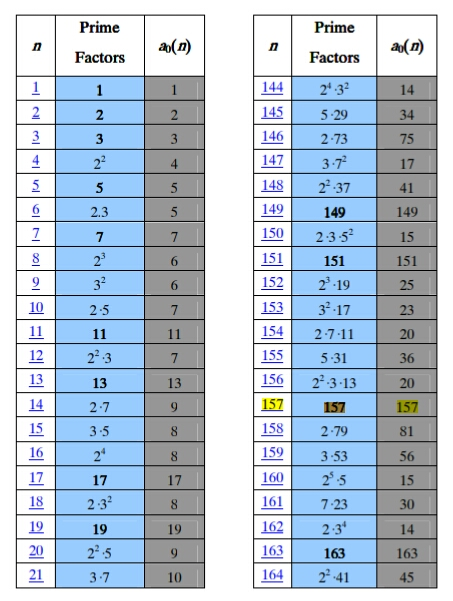By this square correlation between natural and prime numbers then the 571 would be separated by the 100 to 500 and 71 and finally by the form of (2,10) the 500 goes to 50 while 71 is polarized to 71x2=142 and 177 as shown on the table.

(10/2)π = 157 ⇄ (10^2)¹ + 11x7 = 177 = 286 - 109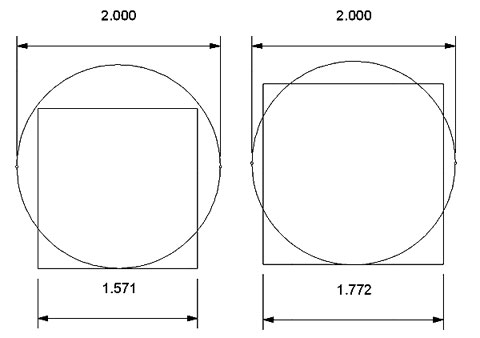So by the above explanation of this 157's behaviour it is now left the question of where the tensor of 571 by the two (2) numbers of 285 and 286 is going. That is the imajinary part (i) of Euler's identity has something to do with the zeta function.

### Encapsulation Scenario

This 4D concept is conducted to get 1000 prime objects of 3rd layer within four (4) times interaction of triangular waves between 26 and 28th prime identities starting from 11x2=22, 22x2=44, 44x2=88 that leads to 88x2=176 objecs of 4th prime identity.

4 x 22 of 88 rows = 4 x 528 = 2112 elements = the index of 1000th prime

``````id: 26
---+-----+-----+-----+-----+
1 |   5 |   1 |  6  |   7 |----------------------------
---+-----+-----+-----+-----+                            |
2 |   2 |   7 |  9  |  16 |----------------------      |
---+-----+-----+-----+-----+                      |     |
3 |  58 |  10 |  68 |  78 |----------------      |     |
---+-----+-----+-----+-----+                |     |     |
4 |  35 |  69 | 104 | 173 |----------      |     |     |
---+-----+-----+-----+-----+          |     |     |     |
5 | {17}| 105 | 122 |{227}|          |     |     |     |
---+-----+-----+-----+-----+- Cross  {17}Δ26|43Δ30|13Δ17|30 ----
6 | {17}|{123}| 140 | 263 |          |     |     |     |       |
---+-----+-----+-----+-----+          |     |     |     |       |
7 |  18 | 141 | 159 | 300 |----------      |     |     |       |
---+-----+-----+-----+-----+                |     |     |       |
8 |  15 | 160 | 175 | 335 |----------------      |     |       |
---+-----+-----+-----+-----+                      |     |       |
9 |  15 | 176 | 191 | 367 |----------------------      |       |
---+-----+-----+-----+-----+                            |       |
10 |  35 |{192}|{227}| 419 |----------------------------        |
---+-----+-----+-----+-----+                                    |
|
id: 27                                                {26}      |
|       |
---+-----+-----+-----+-----+                            |       |
1 |   5 |   1 |   6 |   7 |----------------------    {1+7}     |
---+-----+-----+-----+-----+                      |     |       |
2 |   7 |   7 |  14 |  21 |----------------      |     |       |
---+-----+-----+-----+-----+                |     |   {17}      |
3 |  29 |  15 |  44 |  59 |----------      |     |     |       |
---+-----+-----+-----+-----+          |     |     |     |       |
4 |   8 |  45 |  53 |  98 |          |     |     |     |       |
---+-----+-----+-----+-----+- 4xMEC30 29    2    18 -- Cross -- MEC30
6 |   4 |  54 |  58 | 112 |          |     |     |     |       |
---+-----+-----+-----+-----+          |     |     |     |       |
7 |   - |  59 |  59 | 118 |----------      |     |     |       |
---+-----+-----+-----+-----+                |     |   {17}      |
7 |   9 |  60 |  69 | 129 |----------------      |     |       |
---+-----+-----+-----+-----+                      |     |       |
8 |  23 |  70 |  93 | 163 |----------------------    {1x7}     |
---+-----+-----+-----+-----+                            |       |
|       |
id: 28                                                {28}      |
|
---+-----+-----+-----+-----+                                    |
1 |   5 |  1  |  6  |   7 |----------------------------        |
---+-----+-----+-----+-----+                            |       |
2 |   6 |  7  | 13  |  20 |----------------------      |       |
---+-----+-----+-----+-----+                      |     |       |
3 |   7 | 14  | 21  |  35 |----------------      |     |       |
---+-----+-----+-----+-----+                |     |     |       |
4 |   6 | 22  | 28  |  50 |----------      |     |     |       |
---+-----+-----+-----+-----+          |     |     |     |       |
5 |  13 | 29  | 42  |  71 | Δ13     Δ11   Δ7     0     0 --=---
---+-----+-----+-----+-----+          |     |     |     |
6 |  17 | 43  | 60  | 103 |----------      |     |     |
---+-----+-----+-----+-----+                |     |     |
7 |  14 | 61  | 75  | 136 |----------------      |     |
---+-----+-----+-----+-----+                      |     |
8 |   6 | 76  | 82  | 158 |----------------------      |
---+-----+-----+-----+-----+                            |
9 |   5 | 83  | 88  | 171 |----------------------------
---+-----+-----+-----+-----+
``````

This scheme could be happen by The Encapsulation behaviour of 28 which is the natural number following 27 preceding 29 and depicted as 28 balls arranged in a triangular pattern with the number of layers of 7 which lead to the concept of Gematria.

Twenty-eight is a composite number, its proper divisors being 1, 2, 4, 7, and 14. It is the only known number that can be expressed as a sum of the first nonnegative (or positive) integers ( 0 + 1 + 2 + 3 + 4 + 5 + 6 + 7) and a sum of the first nonprimes ( 1 + 4 + 6 + 8 + 9 ), and it is unlikely that any other number has this property (Wikipedia)..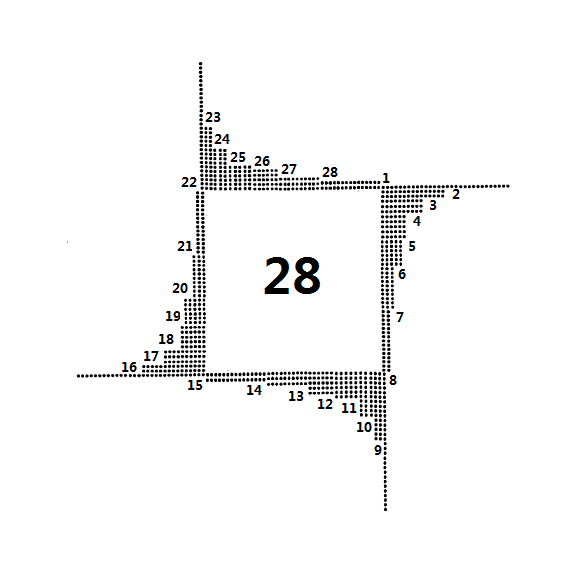However there was a wide discussion stating that Gematria is NOT numerology. impacting a loss of science principal on prime interaction such as in DNA System, which occurs at a mismatch, is said to trigger a shift in the balance, for the binding of the template-primer, from the polymerase, to the exonuclease domain. So it shall use a method that combines data and code.

Encapsulation allows developers to present a consistent and usable interface which is independent of how a system is implemented internally. As one example, encapsulation can be used to hide the values or state of a structured data object inside a class, preventing direct access to them by clients in a way that could expose hidden implementation details or violate state invariance maintained by the methods (Wikipedia).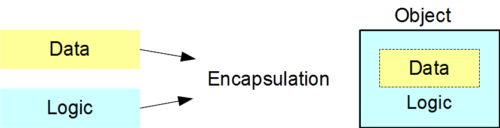Encapsulation is key concept in object-oriented programming (OOP) defined as a way to restrict the direct access to components of an object that users cannot access state values for all of the variables of a particular object. So let's discuss it first.

### Golden Ratio

So it is converting all residual objects out of the prime recycling of Riemann Zeta in to those three (3) basic arithmetic operations of Euler's identity as well the Fibonacy constant (φ) to Euler's number (e). Thus none of residual is neglected by an assumption.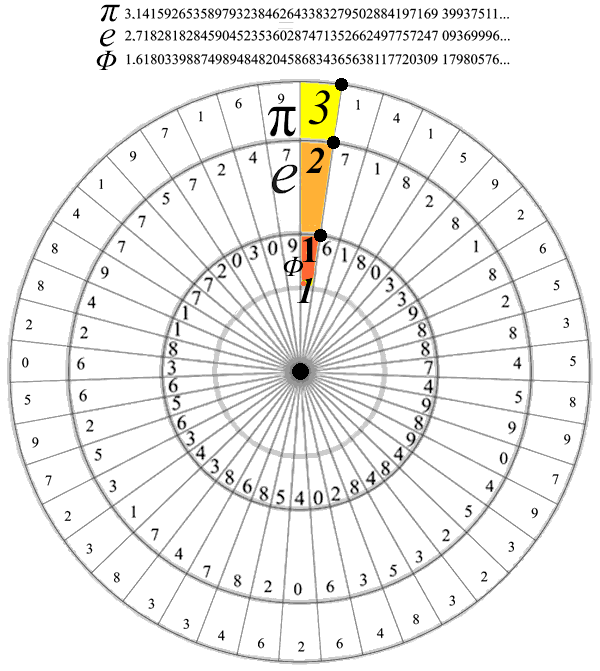This behaviour would come to the feature of golden ratio. However it is not stand as a basic rule but as an impact of 329's vs 289's layers. That is also the reason why we could only see the three (3) digits of 618 out of the Fibonaci constant.

φ = 1.618 = Fibonaci = Golden Ratio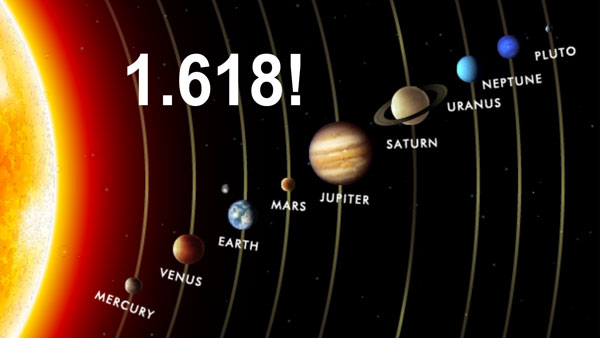Plottng 40th prime scheme of the three (3) layers with all the features of 3rd prime identity as explained above then they would form their recycing through the three (3) times bilateral 9 sums as shown below.

89^2 - 1 = 7920 = 22 x 360 = 66 x 120 = (168 - 102) x 120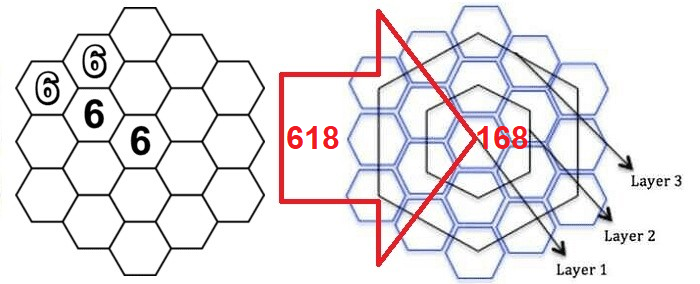``````layer | node | sub |    i     |   f
------+------+-----+----------+-----+-----+-----+                                    ---
|      |     |    1,2:1 |   1 |  30 |  40 | 71 (2,3) ‹-------------------       |
|      |  1  +----------+-----+-----+-----+                              |      |
|  1   |     |        2 |                                                |      5¨  encapsulation
|      |-----+----------+            -----------------------------       |      |
|      |     |        3 |           |                             |      |      |
1   +------+  2  +----------+----       |       LAGGING SCHEME        |      |     ---
|      |     |        4 |           |    (Exponentiation Zone)    |      |      |
|      +-----+----------+           |                             |      |      |
|  2   |     |        5 |           ------------------------------       |      7¨  abstraction
289   |      |  3  +----------+                                                |      |
|     |      |     |        6 |  ‹---------------------------- Φ               | {6®} |
------+------+-----+----------+-----+-----                                     |     ---
|      |     |     11:7 |   5 |   9 |  14 (20) --------› ¤               |      |
|      |  4  +----------+-----+-----+-----+                              |      |
|  3   |     |     12:8 |   9 |  60 |  40 | 109 (26) «------------       |     11¨  polymorphism
|      +-----+----------+-----+-----+-----+                       |      |      |
|      |     |     13:9 |   9 |  60 |  69 (27) «-- Δ19 (Rep Fork) | {2®} |      |
2   +------|  5  +----------+-----+-----+-----+                       |      |     ---
|      |     |    14:19 |   9 |  60 |  40 | 109 (28) -------------       |      |
|      |-----+----------+-----+-----+-----+                              |      |
|  4   |     | 15,18:11 |   1 |  30 |  40 | 71 (29,30,31,32) ------------      13¨  inheritance
329   |      |  6  +----------+-----+-----+-----+                                     |
|     |      |     |    19:12 |  10 |  60 | {70} (36) -------› Φ                      |
------+------+-----+----------+-----+-----+                                          ---
|      |     |    20:13 |  90 |  90 (38) ‹-------------- ¤                      |
|      |  7  +----------+-----+                                                 |
|  5   |     |       14 |            -----------------------------             17¨  class
|      |-----+----------+           |                             |             |
|      |     |       15 |           |       LEADING SCHEME        |             |
3   +------+  8  +----------+-----      |    (Multiplication Zone)    |            ---
|      |     |       16 |           |                             |             |
|      |-----+----------+-----+      -----------------------------              |
|  6   |     |    28:17 | 100 |                                                19¨  object
168   |      |  9  +----------+-----+                                                 |
|     |      |     |    29:18 | 50  | 50(68) ---------> Δ18                           |
------|------|-----+----------+-----+                                                ---
``````

These three (3) times bilateral 9 sums will lead to 168 vs 618 exponents between 68 and 69 objects of 50 and 27th prime identity that simulate the X/Y-genes reproduction of human cromosomes to the repository assignment of our project.

## Registry unit

You can see that two distinct pressure zones are forming and that the spiral pattern expected from lid-driven cavity flow is beginning to form. Experiment with different values of nt to see how long the system takes to stabilize.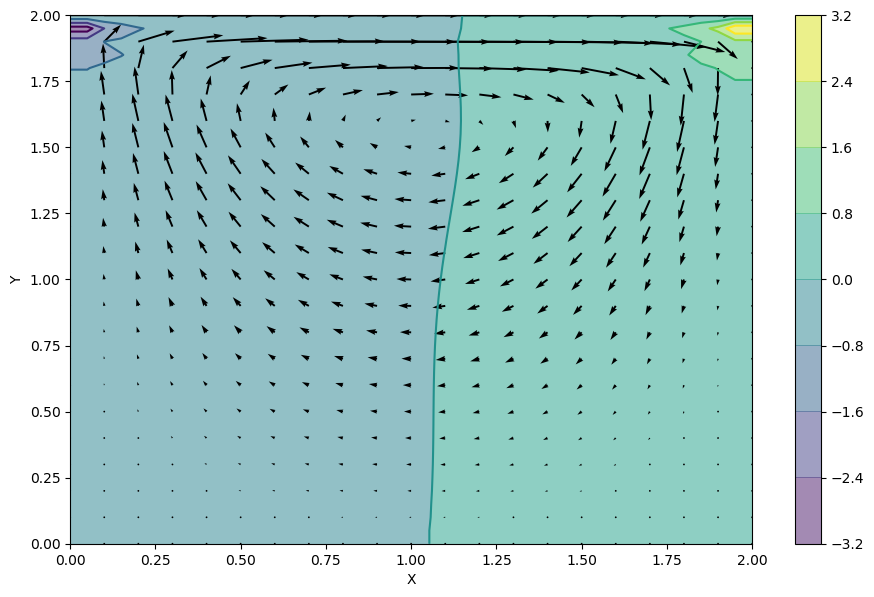It is a relationship between the rate of acceleration of liquids (the increase in their speed) and the force that acts on them, widely used in moving air vehicles, and is considered the most important equation used in the application of aircraft movement.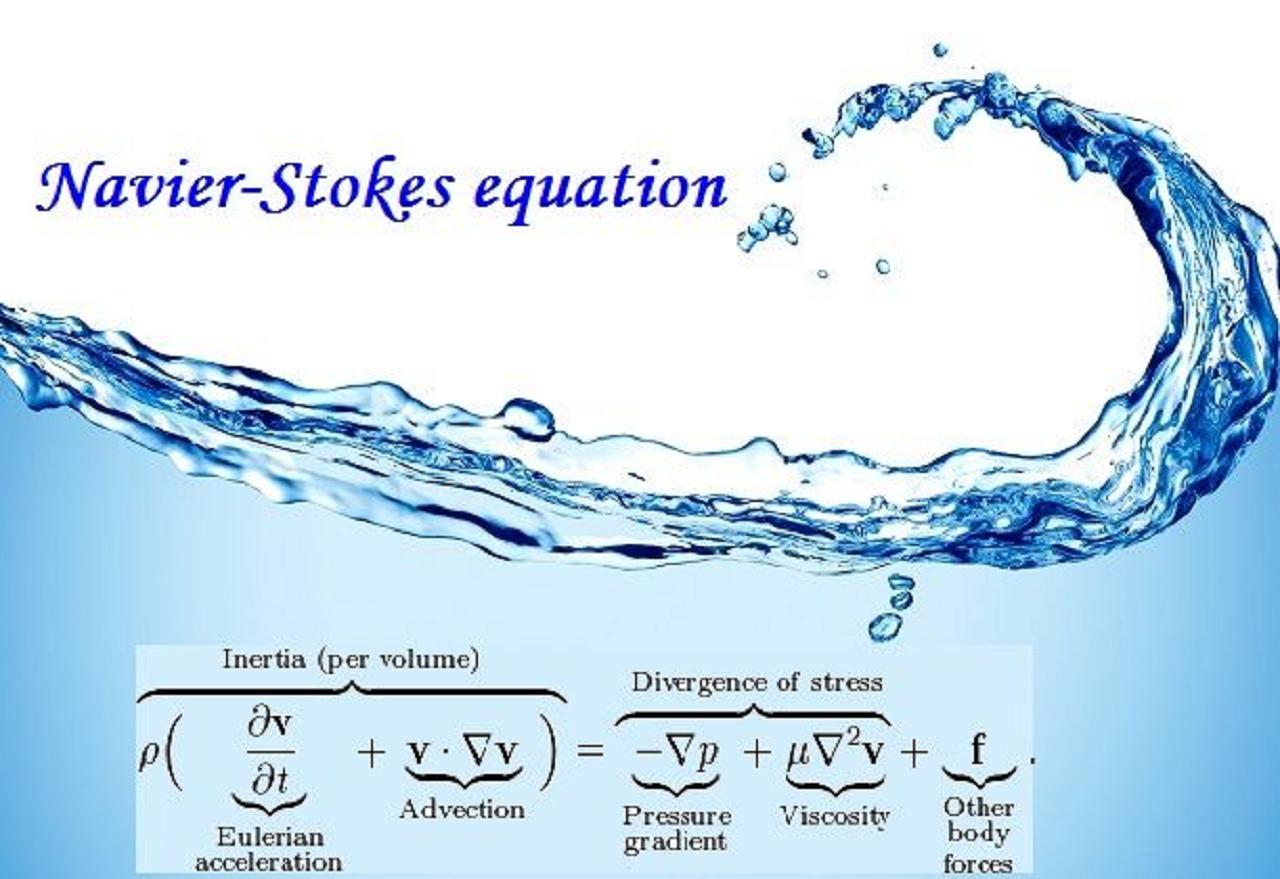It is considered one of the most important equations in physics. Now let's analyze how we could say this structure can be used for switching the workflow between Windows and Linux.